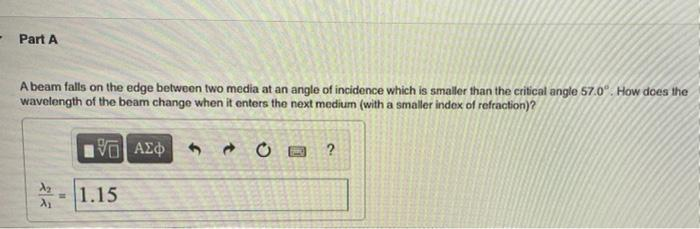# Question Solved1 Answer- Part A A beam falls on the edge between two media at an angle of incidence which is smaller than the critical angle 57.0". How does the wavelength of the beam change when it enters the next medium (with a smaller index of refraction)? O AED 2 ? λα = 1.15 λ,Transcribed Image Text: - Part A A beam falls on the edge between two media at an angle of incidence which is smaller than the critical angle 57.0". How does the wavelength of the beam change when it enters the next medium (with a smaller index of refraction)? O AED 2 ? λα = 1.15 λ,
More
Transcribed Image Text: - Part A A beam falls on the edge between two media at an angle of incidence which is smaller than the critical angle 57.0". How does the wavelength of the beam change when it enters the next medium (with a smaller index of refraction)? O AED 2 ? λα = 1.15 λ,